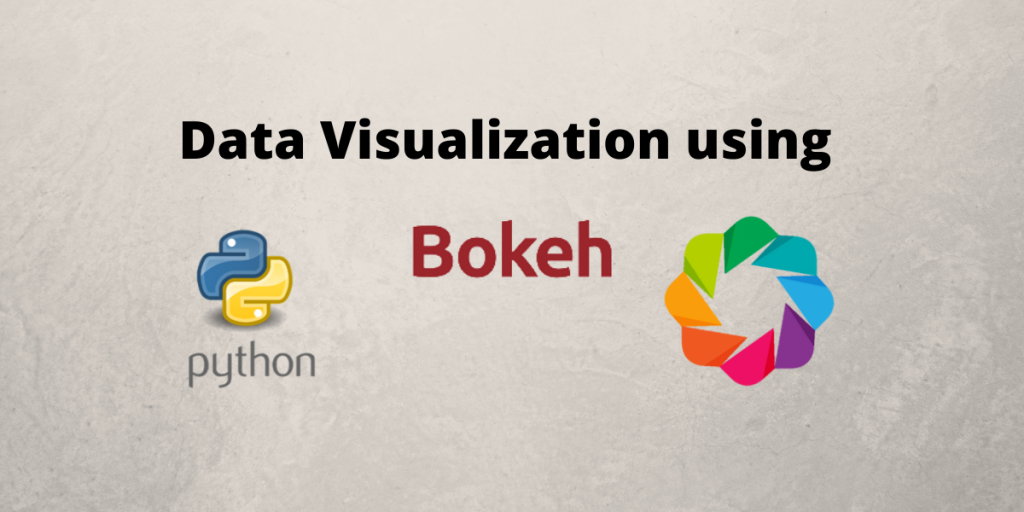# Data Visualization using Python BokehIn this article, we will be looking into data visualization using Python Bokeh.

Bokeh allows users to take in data in any format such as CSV, JSON, hard-coded data, or databases. We can create scatter plots, line charts, etc using this library. It is widely used for stock market analysis in the industry because it is very easy to integrate this library with different web frameworks as well such as JS, Django, and HTML.

## Features of Python Bokeh

1. Interactive: Bokeh is a very interactive library that provides the functionality of interactivity to the graphs in addition to static plots.
2. Powerful: Bokeh is a powerful library because it allows the addition of JavaScript for use-cases.
3. Portable: The output of the Bokeh charts can be rendered on any web framework such as Django and Python and also on Jupyter Notebooks.
4. Flexible: Easy to plot custom and complex use cases.
5. Interaction with other popular tools: Allows easy interaction with pydata tools such as Pandas and Jupyter notebook.

## Starting Out With Data Visualization using Python Bokeh

Whenever we do anything with python, it is a good practice to create a virtual environment and the best way to do is by running the command `pip install pipenv`. Once you run this command, you will have access to the `pipenv` command and you can run the `pipenv shell`. This ensures that the virtual environment is setup.

Now you can use the virtual environment to install Bokeh and Python pandas. You can use the command:

```pipenv install bokeh pandas
```

We will be using pandas because this library allows us to read the CSV file as a dataframe.

### 1. Plotting a line Graph

Before going into plotting charts from the CSV file, we will walk you through the process of plotting a simple line chart to get you familiarized with Bokeh.

```from bokeh.plotting import figure, output_file, show
```
• `figure` module will help users create plots.
• `output_file` will define the name of the HTML file to generate.
• `show` module will generate and show the HTML file.
```x=[1,2,3,4,5]
y=[4,3,3,5,4]
```

For data we just create it as 2 lists -[1,2,3,4,5] and [4,3,3,5,4].

These are points such as (1,4), (2,3), (3,3), and so on.

```output_file('index.html')
```

We set the output file to `index.html` using the above code.

```p = figure(
title = 'Example chart',
x_axis_label = 'X axis',
y_axis_label = 'Y axis'
)
```

We use the figure() to create the plot. The figure() takes in multiple attributes. You can refer to the documentation of this module for further details.

We shall use the `title`, `x_axis_label` and `y_axis_label`.

```p.line(x, y, legend="test", line_width=1.5)
```

Now coming to rendering the glyph, we will use the code snippet above. We specify the two lists `x` and `y` defined earlier. We also specify additional parameters such as `legend` and `line_width`.

Kindly note, these parameters are used here because we are using `line` chart. These parameters tend to vary for the other types of graphs.

```show(p)
```

We use the `show()` function to display the result and the result is displayed on `index.html` as shown below. The entire code is attached as well.

```from bokeh.plotting import figure, output_file, show
x=[1,2,3,4,5]
y=[4,3,3,5,4]

# defining the output file
output_file('index.html')

p = figure(
title = 'Example chart',
x_axis_label = 'X axis',
y_axis_label = 'Y axis'
)

# Rendering the graph
p.line(x, y, legend="test", line_width=1.5)

# Display the results
show(p)
```

### 2. Plotting graphs from CSV files

To plot a graph, we will use a simple dataset of Cars which has 2 columns namely the name of the car and horsepower. We will understand the correlation between these parameters using graphs. The dataset is as shown below

The above dataset can be plotted as a histogram (hbar) graph using Bokeh and the code for this is as follows:

```from bokeh.plotting import figure, output_file, show, save, ColumnDataSource
from bokeh.models.tools import HoverTool
from bokeh.transform import factor_cmap
from bokeh.palettes import Blues8
from bokeh.embed import components
import pandas

source = ColumnDataSource(df)

output_file('index.html')
car_list = source.data['Car'].tolist()

p = figure(
y_range=car_list,
plot_width=800,
plot_height=600,
title='Cars With Top Horsepower',
x_axis_label='Horsepower',
tools="pan,box_select,zoom_in,zoom_out,save,reset"
)

# Render glyph
p.hbar(
y='Car',
right='Horsepower',
left=0,
height=0.4,
fill_color=factor_cmap(
'Car',
palette=Blues8,
factors=car_list
),
fill_alpha=0.9,
source=source,
legend='Car'
)

p.legend.orientation = 'vertical'
p.legend.location = 'top_right'
p.legend.label_text_font_size = '10px'

# Show results
show(p)
```

The output rendered on `index.html` is as follows: